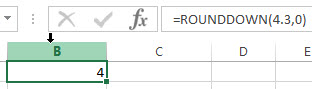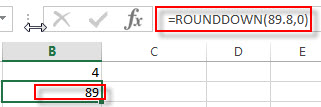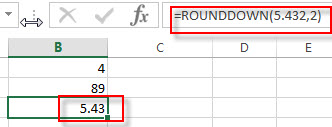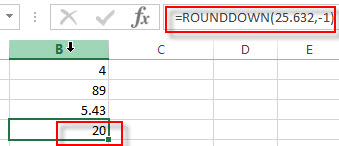# Excel ROUNDDOWN Function

Excel CEILING Function
The Excel CEILING function returns a given number rounded up to the nearest multiple of a given number of significance. The syntax of the CEILING function is as below:= CEILING (number, significance)…This post will guide you how to use Excel ROUNDDOWN function with syntax and examples in Microsoft excel.

### Description

The Excel ROUNDDOWN function round the number down to the specified number of digits. So you can use this function to round a given number down towards zero to a specified number of decimal point.

The ROUNDDOWN function is a build-in function in Microsoft Excel and it is categorized as a Math and Trigonometry Function.

The ROUNDDOWN function is available in Excel 2016, Excel 2013, Excel 2010, Excel 2007, Excel 2003, Excel XP, Excel 2000, Excel 2011 for Mac.

### Syntax

The syntax of the ROUNDDOWN function is as below:

`=ROUNDDOWN (number, num_digits)`

Where the ROUNDDOWN function arguments are:

• number -This is a required argument. Any real number that you want to round down.
• num_digits – This is a required argument. The number of digits to which numbe you wnat to round down.

Note:

• If num_digits argument is greater than 0, then the number is rounded down to the specified number of digits. So it will round down to the right of the decimal point.
• If num_digits argument is equal to 0, then the number is rounded down to the nearest integer.
• If num_digits argument is less than 0, then the number is rounded down to the left of the decimal point.

### Excel ROUNDDOWN Function Examples

The below examples will show you how to use Excel ROUNDDOWN Function to round a given number down to a specified number of decimal point.

1# to round a number 4.3 down to zero decimal places, enter the following formula in Cell B1.

`=ROUNDDOWN(4.3,0)`2# to round a number 89.8 down to zero decimal places, enter the following formula in Cell B2.

`=ROUNDDOWN(89.8,0)`3# to round a number 5.4329 down to two decimal places, enter the following formula in Cell B3.

`=ROUNDDOWN(5.432,2)`4# to round a number 25.632 down to one decimal places to the left of the decimal point, enter the following formula in Cell B4.

`=ROUNDDOWN(25.632,-1)`### Related Functions

• Excel ROUND function
The Excel ROUND function rounds a number to a specified number of digits. You can use the ROUND function to round to the left or right of the decimal point in Excel.The syntax of the ROUND function is as below:=ROUND (number, num_digits)…
• Excel ROUNDUP function
The Excel ROUNDUP function rounds the number up to a specified number of decimal places. It will round away from 0.The syntax of the ROUNDUP function is as below:=ROUNDUP (number, num_digits)…
• Excel CEILING Function
The Excel CEILING function returns a given number rounded up to the nearest multiple of a given number of significance. The syntax of the CEILING function is as below:= CEILING (number, significance)…
• Excel CEILING.PRECISE Function
The Excel CEILING.PRECISE function returns a given number rounded up to the nearest multiple of a given number of significance or returns a number that is rounded up to the nearest integer number.The syntax of the CEILING.PRECISE function is as below:=CEILING.PRECISE (number, [significance])…